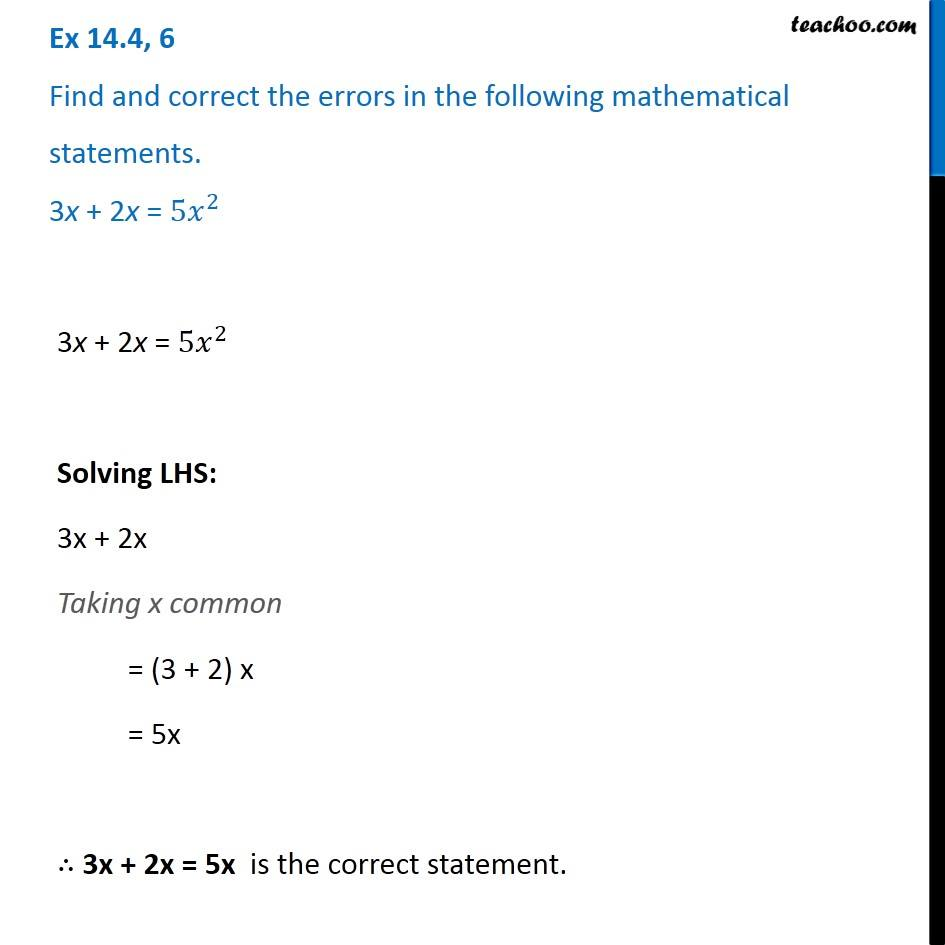Ex 14.4

Chapter 14 Class 8 Factorisation
Serial order wise### Transcript

Ex 14.4, 6 Find and correct the errors in the following mathematical statements. 3x + 2x = 〖5𝑥〗^2 3x + 2x = 〖5𝑥〗^2 Solving LHS: 3x + 2x Taking x common = (3 + 2) x = 5x ∴ 3x + 2x = 5x is the correct statement.